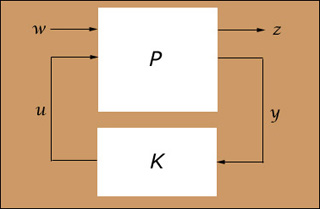6.245 | Spring 2004 | Graduate

# Multivariable Control Systems

## 6-245s04.jpg

Description:

The system above shows a plant (P) which is a finite order linear time-invariant (LTI) feedback system with two inputs, w (disturbance) and u (actuator), two outputs, z (cost) and y (measurement), and a feedback controller (K). (Image courtesy of OCW.)

Alt text:
A diagram of a finite order linear time-invariant (LTI) feedback system.
Caption:
The system above shows a plant (P) which is a finite order linear time-invariant (LTI) feedback system with two inputs, w (disturbance) and u (actuator), two outputs, z (cost) and y (measurement), and a feedback controller (K). (Image courtesy of OCW.)Spring 2004
Lecture Notes